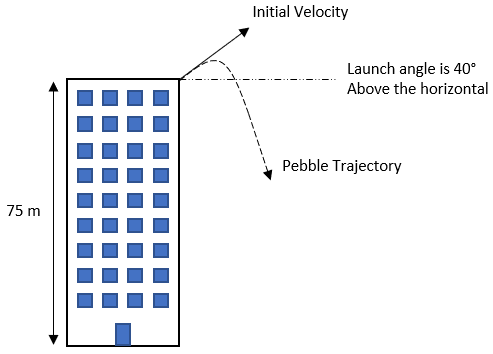## Pebble Off a Building

Consider a pebble is thrown off the roof of a 75 m tall building at 40° above the horizontal. If the initial velocity is 10 m/s, what horizontal distance from the origin will the pebble land on the ground? Ignore friction.Hint
First, calculate the x and y components of the initial velocity:
$$v_x=v_0cos(\theta)$$$$$v_y=v_0sin(\theta)$$$
where $$v_0$$ is initial velocity, and $$\theta$$ is the launch angle above the horizontal in degrees.
Hint 2
Calculate the time it takes the pebble to reach its maximum height:
$$v=at+v_0$$$where $$v$$ is the final velocity, $$a$$ is acceleration due to gravity, $$t$$ is time, and $$v_0$$ is initial velocity. First, calculate the x and y components of the initial velocity: $$v_x=v_0cos(\theta)=(10m/s)cos(40^{\circ})=(10m/s)(0.766)=7.66\:m/s$$$
$$v_y=v_0sin(\theta)=(10m/s)sin(40^{\circ})=(10m/s)(0.643)=6.43\:m/s$$$where $$v_0$$ is initial velocity, and $$\theta$$ is the launch angle above the horizontal in degrees. Next, let’s analyze the pebble’s vertical motion (which involves using $$v_y$$ as the initial velocity). To calculate the time it takes the pebble to reach its maximum height: $$v=at+v_0$$$
where $$v$$ is the final velocity, $$a$$ is acceleration due to gravity, $$t$$ is time, and $$v_0$$ is initial velocity.
$$0m/s=(-9.8m/s^2)t+6.43m/s$$$$$t_{up}=\frac{-6.43m/s}{-9.8m/s^2}=0.66\:sec$$$
To calculate the pebble’s max height from being initially thrown:
$$y=\frac{1}{2}at^2+v_0t+y_0$$$where $$a$$ is the acceleration due to gravity, $$t$$ is the time, $$v_0$$ is the initial velocity, and $$y_0$$ is the initial starting height. Thus, $$y=\frac{1}{2}(-9.8m/s^2)(0.66s)^2+(6.43m/s)(0.66s)+75m$$$
$$y=(-4.9m/s^2)(0.44s^2)+4.22m+75m$$$$$y=-2.16m+4.22m+75m=77\:m$$$
Next, we can reuse the same equation to determine the time it takes the pebble to free fall from maximum height. Since the object is free falling from rest ( $$v_0=0$$ & $$y_0=0$$ ):
$$y=\frac{1}{2}at^2\to 77m=\frac{1}{2}(9.8m/s^2)t^2$$$$$t^2=\frac{(77m)(2)}{9.8m/s^2}=\frac{154}{9.8}s^2=15.7s^2$$$
$$t_{down}=\sqrt{15.7s^2}=3.96\:sec$$$Therefore, the total time the pebble is in the air: $$t_{total}=t_{up}+t_{down}=0.66s+3.96s=4.62\:sec$$$
Looking at motion in the horizontal direction, we can finally solve for the total distance traveled in the x direction:
$$x=v_xt=(7.66m/s)(4.62s)\approx 35\:m$$\$
35 m